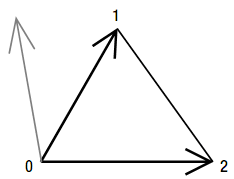# 5.7 自动计算顶点缓冲中所有顶点的法线

## 工作原理

1. 对于结构中的每个顶点，找到使用顶点的三角形。
2. 计算这些三角形的法线向量。
3. 求所有法线向量的平均值。
4. 将这个平均法线存储到顶点中。

1. 对于结构中的每个三角形，计算法线向量。
2. 将这个向量添加到三角形的三个顶点的法线中。对所有三角形进行这个操作后，执行以下操作：
3. 归一化结构中的每个顶点的法线向量。

### 计算三角形的法线：叉乘的定义### GenerateNormalsForTriangleList方法

Private VertexPositionNormalTexture[] GenerateNormalsForTriangleList(VertexPositionNormalTexture[] vertices, int[] indices)
{
} 

### 基于TriangleList计算法线

for (int i = 0; i < vertices.Length; i++)
vertices[i].Normal = new Vector3(0, 0, 0); 

for (int i = 0; i < indices.Length/3; i++)
{
Vector3 firstVec = vertices[indices[i*3 + 1]].Position - vertices[indices[i*3]].Position;
Vector3 secondVec = vertices[indices[i*3 + 2]].Position- vertices[indices[i*3]].Position;
Vector3 normal = Vector3.Cross(secondVec, firstVec);
normal.Normalize();

vertices[indices[i*3]].Normal += normal;
vertices[indices[i*3 + 1]].Normal += normal;
vertices[indices[i*3 + 2]].Normal += normal;
} 

for (int i = 0; i < vertices.Length; i++)
vertices[i].Normal.Normalize();
return vertices;

### 基于TriangleStrip计算法线

for (int i = 2; i < indices.Length; i++)
{
Vector3 firstVec = vertices[indices[i - 1]].Position-vertices[indices[i]].Position;
Vector3 secondVec = vertices[indices[i - 2]].Position-vertices[indices[i]].Position;
Vector3 normal = Vector3.Cross(firstVec, secondVec); normal.Normalize();
} 

bool swappedWinding = false;

for (int i = 2; i < indices.Length; i++)
{
Vector3 firstVec = vertices[indices[i - 1]].Position - vertices[indices[i]].Position;
Vector3 secondVec = vertices[indices[i - 2]].Position -vertices[indices[i]].Position;
Vector3 normal = Vector3.Cross(firstVec, secondVec); normal.Normalize();

if (swappedWinding)
normal *= -1;

vertices[indices[i]].Normal += normal;
vertices[indices[i - 1]].Normal += normal;
vertices[indices[i - 2]].Normal += normal;

swappedWinding = !swappedWinding;
} 

### 使方法Fail-Safe

if (!float.IsNaN(normal.X))
{
vertices[indices[i]].Normal += normal;
vertices[indices[i - 1]].Normal += normal;
vertices[indices[i - 2]].Normal += normal;
} 

### 从顶点缓冲和索引缓冲开始

int numberOfVertices = myVertexBuffer.SizeInBytes / VertexPositionNormalTexture.SizeInBytes;
VertexPositionNormalTexture[] vertices = new VertexPositionNormalTexture[numberOfVertices];
myVertexBuffer.GetData(vertices);

int numberOfIndices = myIndexBuffer.SizeInBytes / 4;
int[] indices = new int[numberOfIndices];
myIndexBuffer.GetData(indices); 

## 代码

private VertexPositionNormalTexture[] GenerateNormalsForTriangleStrip(VertexPositionNormalTexture[] vertices, int[] indices)
{
for (int i = 0; i < vertices.Length; i++)
vertices[i].Normal = new Vector3(0, 0, 0);

bool swappedWinding = false;

for (int i = 2; i < indices.Length; i++)
{
Vector3 firstVec = vertices[indices[i - 1]].Position- vertices[indices[i]].Position;
Vector3 secondVec = vertices[indices[i - 2]].Position - vertices[indices[i]].Position;
Vector3 normal = Vector3.Cross(firstVec, secondVec);
normal.Normalize();

if (swappedWinding)
normal *= -1;

if (!float.IsNaN(normal.X))
{
vertices[indices[i]].Normal += normal;
vertices[indices[i - 1]].Normal += normal;
vertices[indices[i - 2]].Normal += normal;
}

swappedWinding = !swappedWinding;
}

for (int i = 0; i < vertices.Length; i++)
vertices[i].Normal.Normalize();

return vertices;
} 

2006 - 2023，推荐分辨率1024*768以上，推荐浏览器Chrome、Edge等现代浏览器，截止2021年12月5日的访问次数：1872万9823 站长邮箱